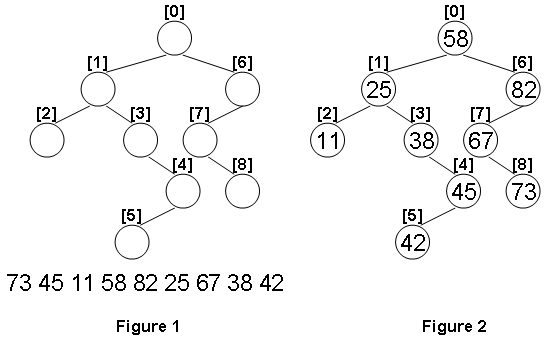# 1099 Build A Binary Search Tree (搜索二叉树赋值并标记左右)

A Binary Search Tree (BST) is recursively defined as a binary tree which has the following properties:
The left subtree of a node contains only nodes with keys less than the node’s key.
The right subtree of a node contains only nodes with keys greater than or equal to the node’s key.
Both the left and right subtrees must also be binary search trees.
Given the structure of a binary tree and a sequence of distinct integer keys, there is only one way to fill these keys into the tree so that the resulting tree satisfies the definition of a BST. You are supposed to output the level order traversal sequence of that tree. The sample is illustrated by Figure 1 and 2.Each input file contains one test case. For each case, the first line gives a positive integer N (<=100) which is the total number of nodes in the tree. The next N lines each contains the left and the right children of a node in the format “left_index right_index”, provided that the nodes are numbered from 0 to N-1, and 0 is always the root. If one child is missing, then -1 will represent the NULL child pointer. Finally N distinct integer keys are given in the last line.

For each test case, print in one line the level order traversal sequence of that tree. All the numbers must be separated by a space, with no extra space at the end of the line.

9
1 6
2 3
-1 -1
-1 4
5 -1
-1 -1
7 -1
-1 8
-1 -1
73 45 11 58 82 25 67 38 42

58 25 82 11 38 67 45 73 42

## 题解

#include<iostream>
#include<algorithm>
using namespace std;
struct node{
long long data,index,l,r,level;
}a;
long long cnt;
long long b;
void def(long long i,long long index,long long level){
if(a[i].l==-1&&a[i].r==-1){
a[i]={b[cnt++],index,a[i].l,a[i].r,level};
}
// 中序遍历从小到大依次赋值给a数组。
else{
// 此处保证左节点肯定比右节点的序号小。
if(a[i].l!=-1)def(a[i].l,index*2+1,level+1);
a[i]={b[cnt++],index,a[i].l,a[i].r,level};
if (a[i].r!=-1) def(a[i].r, index * 2 + 2, level + 1);
}
}
bool cmp(node a,node b){
if(a.level!=b.level)return a.level<b.level;
else return a.index<b.index;
}
int main(){
long long n;
cin>>n;
for(int i=0;i<n;i++)
cin>>a[i].l>>a[i].r;
for(int i=0;i<n;i++)
cin>>b[i];
sort(b,b+n);
def(0,0,0);
sort(a,a+n,cmp);
for(int i=0;i<n;i++)
if(i==0)
cout<<a[i].data;
else cout<<" "<<a[i].data;
return 0;
}

08-01212
02-0714607-06677
08-1495
02-22111
08-111075
03-3180
02-1175
01-2237
03-0161